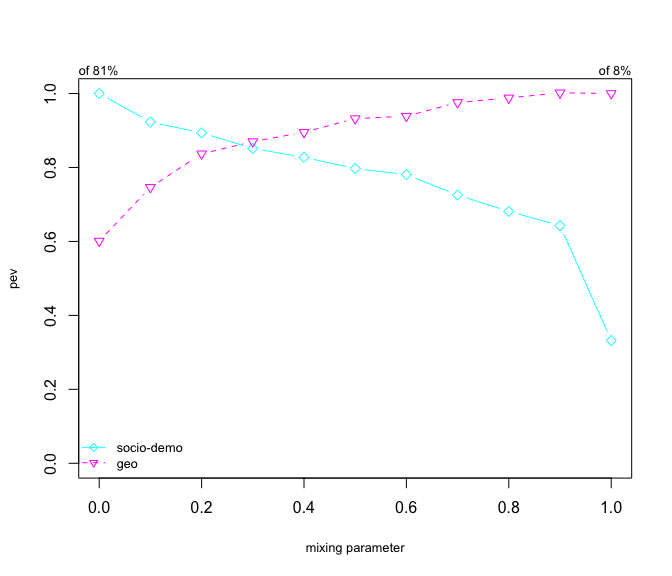# Introduction to Clustgeo

The package Clustgeo is used here for clustering $$n=303$$ french cities. The R dataset estuary is a list of three objects: a matrix dat with the description of the 303 cities on 4 socio-demographic variables, a matrix D.geo with the distances between the town hall of the 303 cities, and an object map of class “SpatialPolygonsDataFrame”.

library(ClustGeo)
data(estuary)
dat <- estuary$dat head(dat) ## employ.rate.city graduate.rate housing.appart agri.land ## 17015 28.08 17.68 5.15 90.04438 ## 17021 30.42 13.13 4.93 58.51182 ## 17030 25.42 16.28 0.00 95.18404 ## 17034 35.08 9.06 0.00 91.01975 ## 17050 28.23 17.13 2.51 61.71171 ## 17052 22.02 12.66 3.22 61.90798 D.geo <- estuary$D.geo
map <- estuary$map ## Ward hierarchical clustering with non euclidean dissimilarity measures and non uniform weights. In this section, we show how to implement and interpret Ward hierarchical clustering when the dissimilarities are not necessary euclidean and the weights are not uniform. We apply first standard hclust function. n <- nrow(dat) D <- dist(dat) Delta <- D^2/(2*n) tree <- hclust(Delta,method="ward.D") We can check that the sum of the heights in hclust’s dendrogram is equal to the total inertia of the dataset if the dissimilarities are euclidean and to the pseudo inertia otherwise. ?inertdiss #pseudo inertia when dissimilarities are non euclidean ?inert #standard inertia otherwise inertdiss(D) #pseudo inertia ##  1232.769 inert(dat) # inertia ##  1232.769 sum(tree$height)
##  1232.769

The same result can be obtained with the function hclustgeo which is a wrapper of hclust taking $$D$$ as input instead of $$\Delta$$.

tree <- hclustgeo(D)
sum(tree$height) ##  1232.769 When the weights are not uniform, the calculation of the matrix $$\Delta$$ takes a few lines of code and the use of the function hclustgeo may be more convenient than hclust. map <- estuary$map
wt <- [email protected]$POPULATION # non uniform weights # with hclust Delta <- D for (i in 1:(n-1)) { for (j in (i+1):n) { Delta[n*(i-1) - i*(i-1)/2 + j-i] <- Delta[n*(i-1) - i*(i-1)/2 + j-i]^2*wt[i]*wt[j]/(wt[i]+wt[j]) }} tree <- hclust(Delta,method="ward.D",members=wt) sum(tree$height)
##  1907989
#with hclustgeo
tree <- hclustgeo(D,wt=wt)
sum(tree$height) ##  1907989 ## Ward-like hierarchical clustering with geographical constraints. Now we consider two dissimilarity matrices : • $$D_0$$ is the euclidean distance matrix performed with the socio-demographic variables • $$D_1$$ is a second dissimilarity matrix used to take the geographical proximity between the cities into account. The function hclustgeo implements a Ward-like hierarchical clustering algorithm including soft contiguity constraints. This algorithm takes as input two dissimilarity matrices D0 and D1 and a mixing parameter $$\alpha$$ between 0 an 1. The first matrix gives the dissimilarities in the “feature space” (here socio-demographic variables). The second matrix gives the dissimilarities in the “constraint” space (here the matrix of geographical distances or the matrix build from the contiguity matrix $$C$$). The mixing parameter $$\alpha$$ sets the importance of the constraint in the clustering procedure. We present here a procedure to choose the mixing parameter $$\alpha$$ with the function choicealpha. ### Choice of a partition with $$D_0$$ only First, we choose $$K=5$$ clusters from the Ward dendrogram obtained with the socio-demographic variables ($$D_0$$) only. library(ClustGeo) data(estuary) dat <- estuary$dat
D.geo <- estuary$D.geo map <- estuary$map

D0 <- dist(dat) # the socio-demographic distances
tree <- hclustgeo(D0)
plot(tree,hang=-1,label=FALSE, xlab="",sub="",
main="Ward dendrogram with D0 only",cex.main=0.8,cex=0.8,cex.axis=0.8,cex.lab=0.8)
#plot(tree,hang=-1,xlab="",sub="",main="Ward dendrogram with D0 only",
#      cex.main=0.8,cex=0.8,labels=city_label,cex.axis=0.8,cex.lab=0.8)
rect.hclust(tree,k=5,border=c(4,5,3,2,1))
legend("topright", legend= paste("cluster",1:5), fill=1:5, cex=0.8,bty="n",border="white")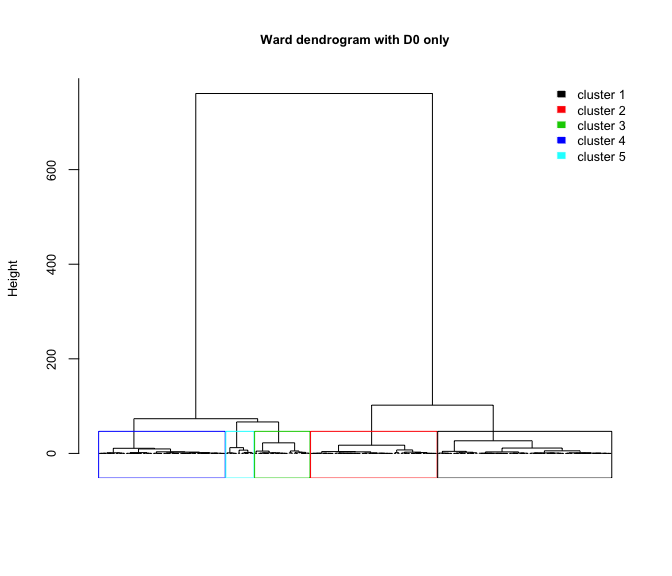We can use the map given with the estuary data.

# the map of the cities is an object of class "SpatialPolygonsDataFrame"
class(map)
##  "SpatialPolygonsDataFrame"
## attr(,"package")
##  "sp"
# the object map contains several informations
names(map)
##   "ID_GEOFLA"  "CODE_COMM"  "INSEE_COM"  "NOM_COMM"   "X_CHF_LIEU"
##   "Y_CHF_LIEU" "X_CENTROID" "Y_CENTROID" "Z_MOYEN"    "SUPERFICIE"
##  "POPULATION" "CODE_CANT"  "CODE_ARR"   "CODE_DEPT"  "NOM_DEPT"
##  "CODE_REG"   "NOM_REGION" "AREA_HA"
head([email protected][,4:8])
##        NOM_COMM X_CHF_LIEU Y_CHF_LIEU X_CENTROID Y_CENTROID
## 17015     ARCES       3989      65021       3979      65030
## 17021    ARVERT       3791      65240       3795      65247
## 17030  BALANZAC       4018      65230       4012      65237
## 17034    BARZAN       3991      64991       3982      64997
## 17050      BOIS       4189      64940       4176      64934
## 17052 BOISREDON       4227      64745       4224      64749
# we check that the cities in map are the same than those in X
identical(as.vector(map$"INSEE_COM"),rownames(dat)) ##  TRUE # now we plot the cities on the map with the name of four cities city_label <- as.vector(map$"NOM_COMM")
sp::plot(map,border="grey")
text(sp::coordinates(map)[c(54,99,117,116),],labels=city_label[c(54,99,117,116)],cex=0.8)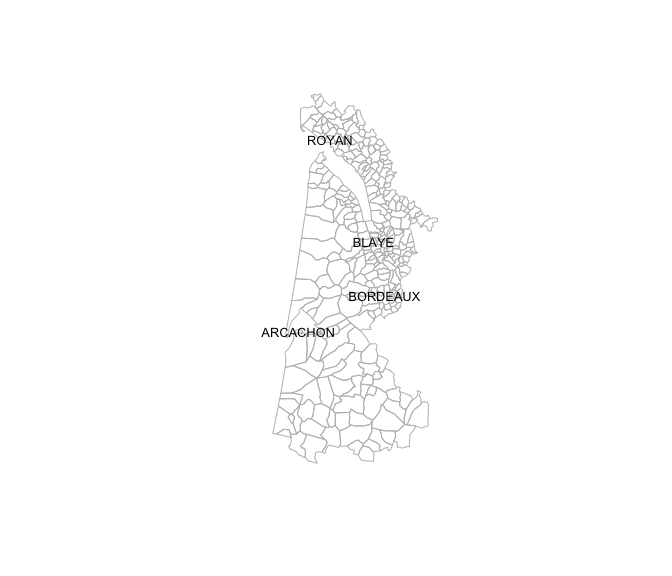Let us plot these 5 clusters on the map.

# cut the dendrogram to get the partition in 5 clusters
P5 <- cutree(tree,5)
names(P5) <- city_label
sp::plot(map,border="grey",col=P5,main="5 clusters partition obtained with D0 only",cex.main=0.8)
legend("topleft", legend=paste("cluster",1:5), fill=1:5, cex=0.8,bty="n",border="white")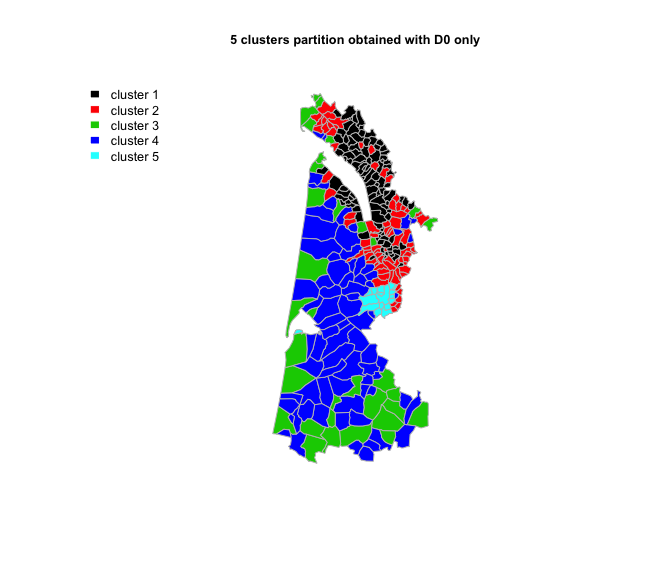We can notice that the cities in the cluster 5 are geographically very homogeneous.

# list of the cities in cluster 5
city_label[which(P5==5)]
##   "ARCACHON"          "BASSENS"           "BEGLES"
##   "BORDEAUX"          "LE BOUSCAT"        "BRUGES"
##   "CARBON-BLANC"      "CENON"             "EYSINES"
##  "FLOIRAC"           "GRADIGNAN"         "LE HAILLAN"
##  "LORMONT"           "MERIGNAC"          "PESSAC"
##  "TALENCE"           "VILLENAVE-D'ORNON"
#plot(map,border="grey")
#text(coordinates(map)[which(P5==5),],labels=city_label[which(P5==5)],cex=0.8)

On the contrary the cities in cluster 3 are geographically very splitted.

### Change the partition to take geographical constraint into account

In order to get more geographically compact clusters, we introduce now the matrix $$D_1$$ of the geographical distances in hclustgeo.

D1 <- as.dist(D.geo) # the geographic distances between the cities
# 

For that purpose, we have to choose a mixing parameter $$\alpha$$ to improve the geographical cohesion of the 5 clusters of the partition found previously without deteriorating the socio-demographic cohesion too much.

#### Choice of the mixing parameter $$\alpha$$

The mixing parameter $$\alpha \in [0,1]$$ sets the importance of $$D_0$$ and $$D_1$$ in the clustering process. When $$\alpha=0$$ the geographical dissimilarities are not taken into account and when $$\alpha=1$$ it is the socio-demographic distances which are not taken into account and the clusters are obtained with the geographical distances only.

The idea is then to calculate separately the socio-demographic homogeneity and the geographic cohesion of the partitions obtained for a range of different values of $$\alpha$$ and a given number of clusters $$K$$.

The idea is to plot the quality criterion $$Q_O$$ and $$Q_1$$ of the partitions $$P_K^\alpha$$ obtained with different values of $$\alpha \in [0,1]$$ and to choose the value of $$\alpha$$ which is a compromise between the lost of socio-demographic homogeneity and the gain of geographic cohesion. We use the function choicealpha for that purpose.

range.alpha <- seq(0,1,0.1)
K <- 5
cr <- choicealpha(D0,D1,range.alpha,K,graph=TRUE)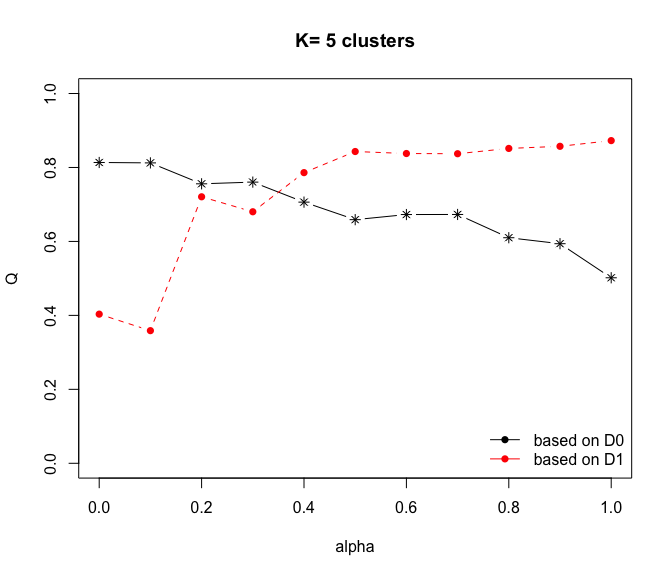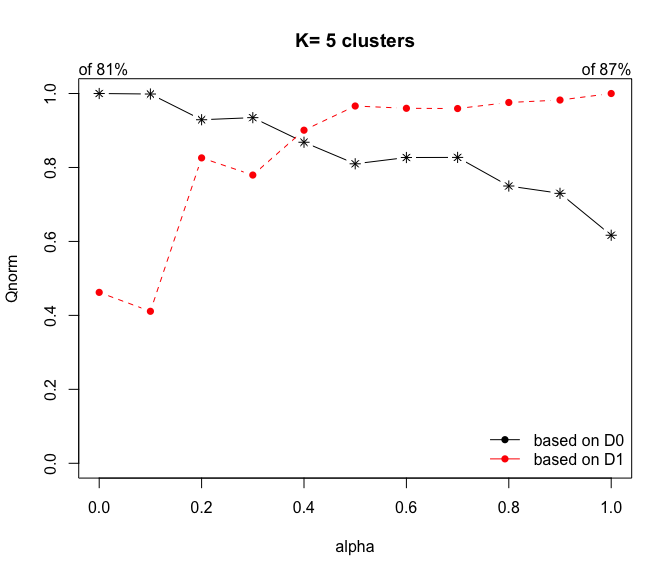cr$Q # proportion of explained pseudo inertia ## Q0 Q1 ## alpha=0 0.8134914 0.4033353 ## alpha=0.1 0.8123718 0.3586957 ## alpha=0.2 0.7558058 0.7206956 ## alpha=0.3 0.7603870 0.6802037 ## alpha=0.4 0.7062677 0.7860465 ## alpha=0.5 0.6588582 0.8431391 ## alpha=0.6 0.6726921 0.8377236 ## alpha=0.7 0.6729165 0.8371600 ## alpha=0.8 0.6100119 0.8514754 ## alpha=0.9 0.5938617 0.8572188 ## alpha=1 0.5016793 0.8726302 cr$Qnorm # normalized proportion of explained pseudo inertia
##              Q0norm    Q1norm
## alpha=0   1.0000000 0.4622065
## alpha=0.1 0.9986237 0.4110512
## alpha=0.2 0.9290889 0.8258889
## alpha=0.3 0.9347203 0.7794868
## alpha=0.4 0.8681932 0.9007785
## alpha=0.5 0.8099142 0.9662043
## alpha=0.6 0.8269197 0.9599984
## alpha=0.7 0.8271956 0.9593526
## alpha=0.8 0.7498689 0.9757574
## alpha=0.9 0.7300160 0.9823391
## alpha=1   0.6166990 1.0000000
?plot.choicealpha
#plot(cr,norm=TRUE)

We see on the plot the proportion of explained pseudo inertia calculated with $$D_0$$ (the socio-demographic distances) is equal to 0.81 when $$\alpha=0$$ and decreases when $$\alpha$$ inceases (black line). On the contrary the proportion of explained pseudo inertia calculated with $$D_1$$ (the socio-demographic distances) is equal to 0.87 when $$\alpha=1$$ and decreases when $$\alpha$$ decreases (red line).

Here the plot suggest to choose $$\alpha=0.2$$ which correponds to a lost of socio-demographic homogeneity of only 7 % and a gain of geographic homogeneity of about 17 %.

#### Modified partition obtained with $$\alpha=0.2$$.

We perform hclustgeo with $$D_0$$ and $$D_1$$ and $$\alpha=0.2$$ and cut the tree to get the new partition in 5 clusters.

tree <- hclustgeo(D0,D1,alpha=0.2)
P5bis <- cutree(tree,5)

The gain in geographic cohesion of this partition can also be visualized on the map.

tree <- hclustgeo(D0,D1,alpha=0.2)
P5bis <- cutree(tree,5)
sp::plot(map,border="grey",col=P5bis, main="5 clusters partition obtained \n with alpha=0.2 and geographical distances",cex.main=0.8)
legend("topleft", legend=paste("cluster",1:5), fill=1:5, bty="n",border="white")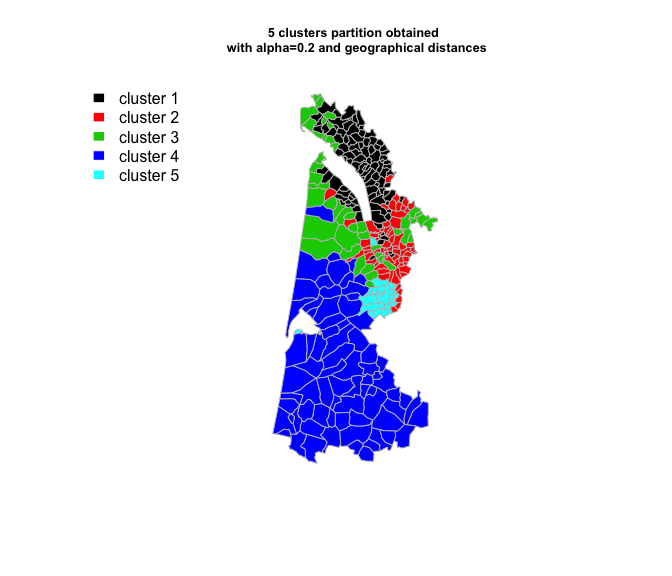### Change the partition to take neighborhood constraint into account

Let us construct a different type of matrix $$D_1$$ to take the neighborhood between the regions into account for clustering the 303 cities.
Two regions with contiguous boundaries, that is sharing one or more boundary point are considered as neighbours. Let us first build the adjacency matrix $$A$$.

#library(spdep)
list.nb <- spdep::poly2nb(map,row.names = rownames(dat)) #list of neighbours of each city
city_label[list.nb[]] # list of the neighbours of BORDEAUX
##   "BASSENS"     "BEGLES"      "BLANQUEFORT" "LE BOUSCAT"  "BRUGES"
##   "CENON"       "EYSINES"     "FLOIRAC"     "LORMONT"     "MERIGNAC"
##  "PESSAC"      "TALENCE"
A <- spdep::nb2mat(list.nb,style="B")
diag(A) <- 1
colnames(A) <- rownames(A) <- city_label
A[1:5,1:5]
##          ARCES ARVERT BALANZAC BARZAN BOIS
## ARCES        1      0        0      1    0
## ARVERT       0      1        0      0    0
## BALANZAC     0      0        1      0    0
## BARZAN       1      0        0      1    0
## BOIS         0      0        0      0    1

The dissimilarity matrix $$D_1$$ is build from the adjacency matrix $$A$$ with $$D_1=1-A$$.

D1 <- as.dist(1-A)

#### Choice of the mixing parameter $$\alpha$$

The same procedure for the choice of $$\alpha$$ is then used with this neighborhood dissimilarity matrix $$D_1$$.

cr <- choicealpha(D0,D1,range.alpha,K,graph=TRUE)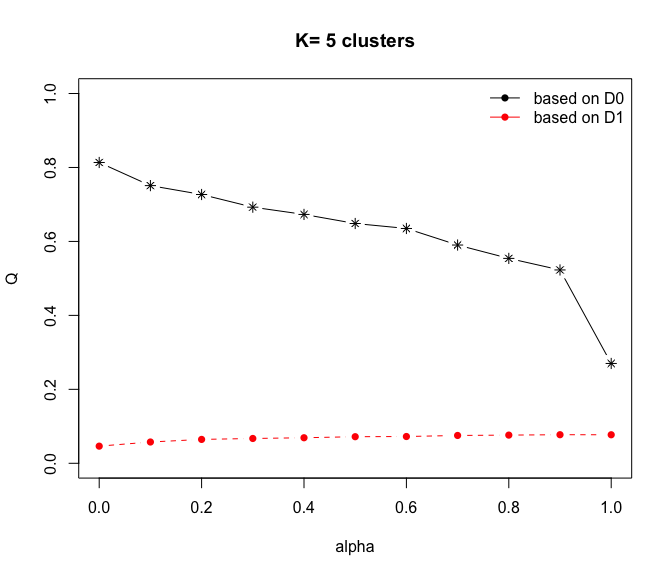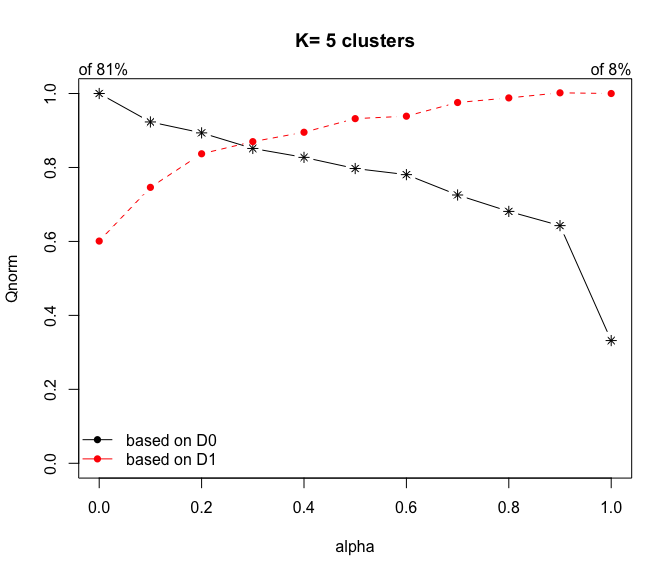cr$Q # proportion of explained pseudo inertia ## Q0 Q1 ## alpha=0 0.8134914 0.04635748 ## alpha=0.1 0.7509422 0.05756315 ## alpha=0.2 0.7268960 0.06456769 ## alpha=0.3 0.6926275 0.06710020 ## alpha=0.4 0.6730000 0.06905647 ## alpha=0.5 0.6484593 0.07190000 ## alpha=0.6 0.6350298 0.07240719 ## alpha=0.7 0.5902430 0.07526225 ## alpha=0.8 0.5541168 0.07622149 ## alpha=0.9 0.5228693 0.07728260 ## alpha=1 0.2699756 0.07714010 cr$Qnorm # normalized proportion of explained pseudo inertia
##              Q0norm    Q1norm
## alpha=0   1.0000000 0.6009518
## alpha=0.1 0.9231102 0.7462157
## alpha=0.2 0.8935510 0.8370184
## alpha=0.3 0.8514257 0.8698485
## alpha=0.4 0.8272982 0.8952084
## alpha=0.5 0.7971311 0.9320703
## alpha=0.6 0.7806226 0.9386452
## alpha=0.7 0.7255676 0.9756566
## alpha=0.8 0.6811587 0.9880916
## alpha=0.9 0.6427471 1.0018473
## alpha=1   0.3318727 1.0000000
tree <- hclustgeo(D0,D1,alpha=0.2)
P5ter <- cutree(tree,5)
sp::plot(map,border="grey",col=P5ter, main="5 clusters partition obtained with \n alpha=0.2 and neighbours dissimilarities",cex.main=0.8)
legend("topleft", legend=paste("cluster",1:5), fill=1:5, bty="n",border="white",cex=0.8)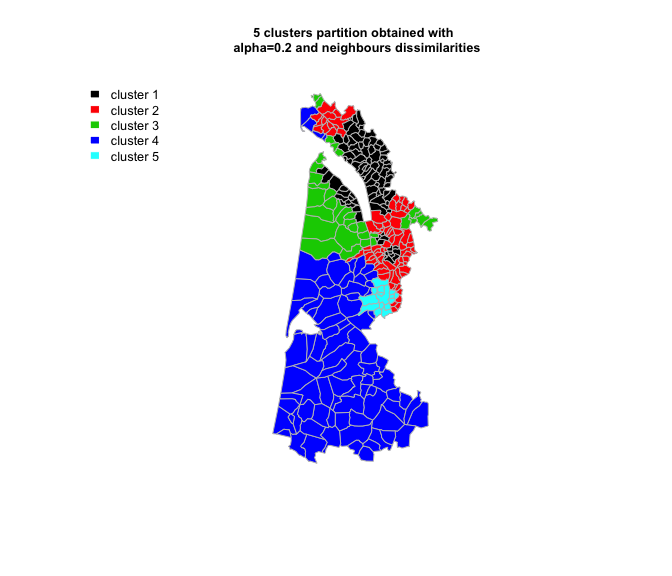With this kind of local dissimilaritis in $$D_1$$, the neighborhood within cohesion is always very small. To overcome this problem, we can use the plot the normalized proportion of explained inertia ($$Qnorm$$) instead the proportion of explained inertia ($$Q$$). The plot of $$Qnorm$$ suggest again $$\alpha=0.2$$.

#### Modified partition obtained with $$\alpha=0.2$$.

tree <- hclustgeo(D0,D1,alpha=0.2)
P5bis <- cutree(tree,5)
sp::plot(map,border="grey",col=P5bis, main="5 clusters partition obtained with \n alpha=0.2 and neighborhood dissimilarities",cex.main=0.8)
legend("topleft", legend=1:5, fill=1:5, col=P5,cex=0.8)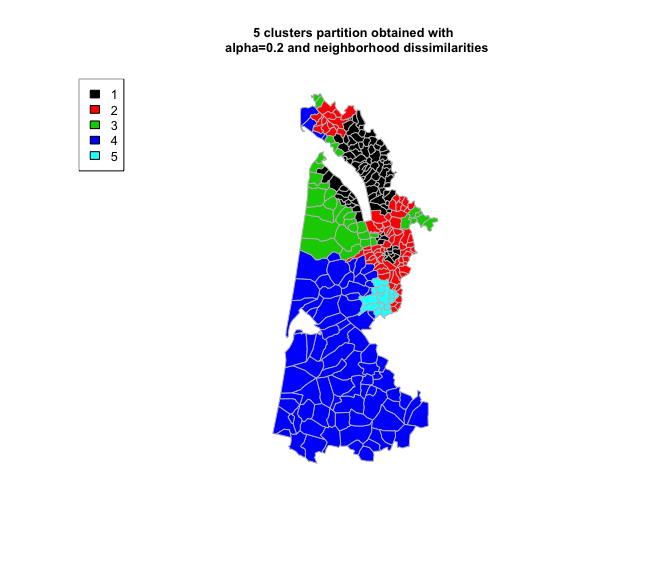### The method plot.choicealpha

These plots can be obtained with the plot method plot.choicealpha.

range.alpha <- seq(0,1,0.1)
K <- 5
cr <- choicealpha(D0,D1,range.alpha,K,graph=FALSE)
?plot.choicealpha
plot(cr,cex=0.8,norm=FALSE,cex.lab=0.8,ylab="pev",col=3:4,legend=c("socio-demo","geo"),
xlab="mixing parameter")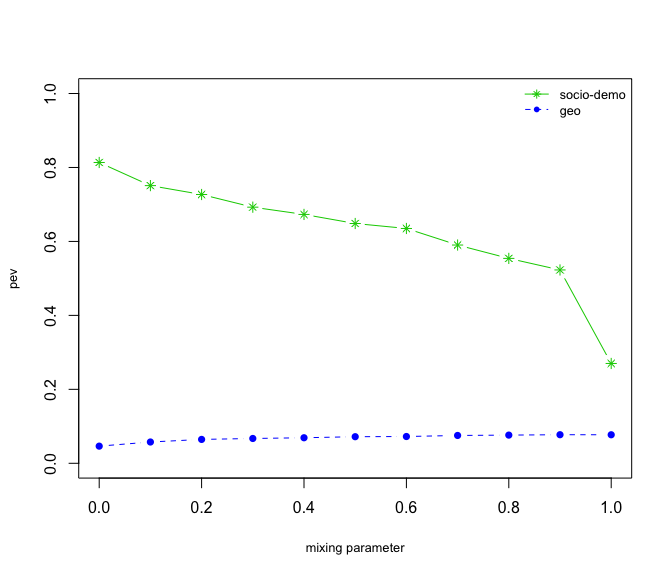plot(cr,cex=0.8,norm=TRUE,cex.lab=0.8,ylab="pev",col=5:6,pch=5:6,legend=c("socio-demo","geo"),
xlab="mixing parameter")